# To estimate: The value of f ′ ( 0 ) , f ′ ( 1 2 ) , f ′ ( 1 ) , f ′ ( 2 ) and f ′ ( 3 ) by using a graphing device to zoom in on the graph of f.### Single Variable Calculus: Concepts...

4th Edition
James Stewart
Publisher: Cengage Learning
ISBN: 9781337687805### Single Variable Calculus: Concepts...

4th Edition
James Stewart
Publisher: Cengage Learning
ISBN: 9781337687805

#### Solutions

Chapter 2.7, Problem 18E

(a)

To determine

## To estimate: The value of f′(0), f′(12), f′(1), f′(2) and f′(3) by using a graphing device to zoom in on the graph of f.

Expert Solution

The value of f(0), f(12), f(1), f(2) and f(3) are 0, 0.75, 3, 12 and 27.

### Explanation of Solution

Given:

The function is f(x)=x3.

Estimation:

Obtain f(x) at the point 0.

Use the online graphing calculator to zoom toward the point (0,0) as shown below in Figure 1.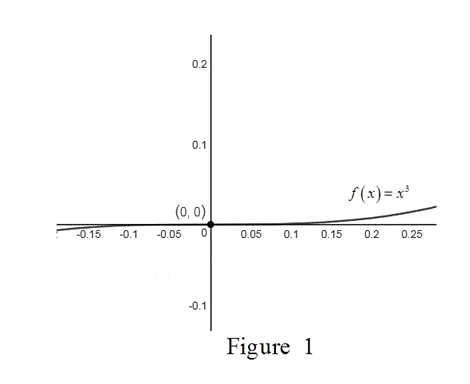The calculation of f(0) is as follows,

From Figure 1, the tangent to the curve at x=0 is the x-axis.

So, f(0)=0.

Thus, f(0)=0.

Obtain f(x) at the point 12.

Use the online graphing calculator to zoom toward the point (12,18) as shown below in Figure 2.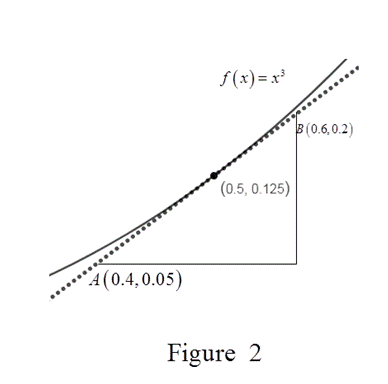The calculation of f(12) is as follows,

From Figure 2,the slope of the tangent is as follows,

m=0.050.20.40.6=0.150.2=1520=0.75

Thus, f(12)=0.75.

Obtain f(x) at the point 1.

Use the online graphing calculator to zoom toward the point (1,1) as shown below in Figure 3.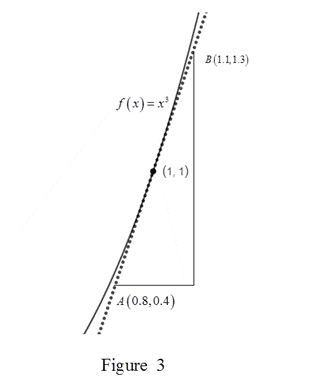The calculation of f(1) is as follows.

From Figure 3, the slope of tangent is,

m=0.41.30.81.1=0.90.3=3

Thus, f(1)=3.

Obtain f(x) at the point 2.

Use the online graphing calculator to zoom toward the point (2,8) as shown below in Figure 4.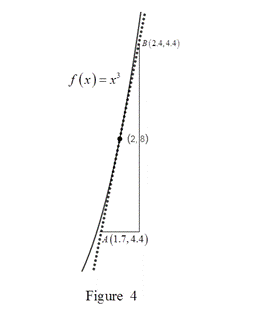The calculation of f(2) is as follows.

From Figure 4,the slope of tangent is

m=4.412.81.72.4=8.40.7=12

Thus, f(2)=12.

Obtain f(x) at the point 3.

Use the online graphing calculator to zoom toward the point (3,27) as shown below in Figure 4.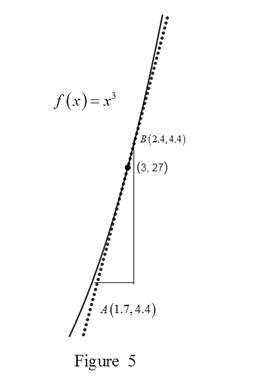The calculation of f(3) is as follows,

From Figure 5, the slope of tangent is

m=43.216.23.62.6=271=27

Thus, f(3)=27.

(b)

To determine

### To deduce: The values of f′(−12),f′(−1),f′(−2) and f′(−3) using symmetry.

Expert Solution

The values of f(12),f(1),f(2) and f(3) are 0.75, 3, 12 and 27 respectively.

### Explanation of Solution

Result Used:

For any odd and even function,

f(x)=f(x) and f(x)=f(x) holds respectively.

Calculation:

The function f(x)=x3 is odd function.

Thus, the function f(x) is an even function.

From part (a),

f(12)=0.75,f(1)=1,f(2)=12 and f(3)=27.

Using the symmetry,

f(12)=f(12)=0.75

Thus, f(12)=0.75.

Using the symmetry,

f(1)=f(1)=3

Thus, f(1)=3.

Using the symmetry,

f(2)=f(2)=12

Thus, f(2)=12.

Using the symmetry,

f(3)=f(3)=27

Thus, f(3)=27.

(c)

To determine

Expert Solution

### Explanation of Solution

Graph:

Use the information from part a and b and sketch the graph of f as shown in Figure 6.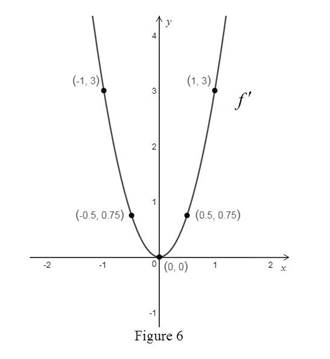From Figure 6, it is observed that the function f is an even function.

(d)

To determine

### To guess: The formula of f′ by using part a and part b.

Expert Solution

The formula of f is f(x)=3x2

### Explanation of Solution

From part a and part b,

f(12)=34=3(14)=3(12)2

f(1)=3=3(1)2

f(2)=12=3(4)=3(2)2

f(3)=27=3(9)=3(3)2

It is observed from the above calculations that the derivative is thrice the square of the input value.

Thus, the formula for the function is, f(x)=3x2.

(e)

To determine

Expert Solution

### Explanation of Solution

Definition used:

The derivation of a function is given by the formula,

f(x)=limh0f(x+h)f(x)h

Proof:

Consider the function, f(x)=x3.

Use the definition of derivative to obtain the derivative of f(x)=x3.

f(x)=limh0f(x+h)f(x)h=limh0(x+h)3(x)3h=limh0x3+h3+3x2h+3xh2x3h

Simplify the terms in numerator,

f(x)=limh0h3+3x2h+3xh2h

Since the limit h approaches zero but not equal to zero, cancel the common term h from both the numerator and the denominator,

f(x)=limh0(h2+3x2+3xh)=limh0(h2)+3x2limh0(1)+3xlimh0(h)=0+3x2+3x(0)=3x2

So, f(x)=3x2.

Thus, the guess in part d is correct.

### Have a homework question?

Subscribe to bartleby learn! Ask subject matter experts 30 homework questions each month. Plus, you’ll have access to millions of step-by-step textbook answers!